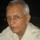Coffee Room
Discuss anything here - everything that you wish to discuss with fellow engineers.
12763 Members
Join this group to post and comment.Aruwin • Feb 12, 2012

Conditional extreme values

Can there be two maximum points of a function with 2 variables?
For example, this question:
Find the extreme values (state whether it's maximum or minimum) of f(x,y)= x^2 + 2xy + 7y^2 under the terms of x^2 + 4y^2 = 5

I got 2 minimum values at f(1,1)= 10 and at f(-1,-1)=10. Turns out that the values at both points are the same. Can this be correct?

Here's my working:

Let f(x,y)= x^2 + 2xy + 7y^2 with constraint g(x,y) = x^2 + 4y^2 = 5.
Then, ∇f = λ∇g ==> <2x + 2y, 2y + 14y> = λ<2x, 8y>.

Equate like entries:
2x + 2y = 2λx ==> x + y = λx
2y + 14y = 2λy ==> 2 = λ

So, x + y = λx
==> y = x.

Substitute this into g ==> x^2+4x^2=5 and thus, x= 1 or -1
Hence, (x,y) = (1,1) and (-1,-1)

D(1,1) = (-2)*(32) + (-14)*(16)= -288 <0
D(-1,-1)=(-2)*(32)+(-4)(0)=-64<0

SO,the maximum value at f(1,1)=10 and at f(-1,-1)=10​Aruwin
Can there be two maximum points of a function with 2 variables?
I am not quite sure if this is relevant to the question.

Consider the sine function: there are infinite number of maxima and minima.
Consider a modulated sine wave:You have an infinite number of maxima and minima. These themselves form sine waves, with its own maxima and minima.Aruwin • Feb 12, 2012
bioramani
I am not quite sure if this is relevant to the question.

Consider the sine function: there are infinite number of maxima and minima.
Consider a modulated sine wave:You have an infinite number of maxima and minima. These themselves form sine waves, with its own maxima and minima.
So it's alright then. But is my answer correct??Is the maximum point 10?